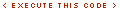# Fx.Tween

Here is the documentation for Fx.Tween.js.

This is where MooTools really shines. Smoothly modifying the properties of an element is super simple. You have a couple of options on how to do it, once again, and they each have trade offs. I'll start with the method of creating an object for the effect:

```/*	you should use "var exampleFx = ..." below
but due to the way I execute code in these blocks,
I declared this variable already */
exampleFx = new Fx.Tween('fxTarget', {
property: 'opacity',
duration: 500,
transition: Fx.Transitions.Quart.easeInOut
});
/*	now we have an fx object, let's play with it:	*/

exampleFx.start.pass([0,.5], exampleFx).delay(1000);
/*	wait a sec, bring it back to half way
then wait another sec and bring it all the way back	*/
exampleFx.start.pass([.5,1], exampleFx).delay(2000);
/*	let's try from visible to hidden then back	*/
exampleFx.start.pass([1,0], exampleFx).delay(3000);
exampleFx.start.pass([0,1], exampleFx).delay(4000);```In the example above, I'm calling the start function which will alter the property I declared when I created the effect (in this instance, opacity). The effect will translate that property from the start to end values I pass in with the start function for the duration I specify (the default duration is 500ms).

Also, the example above shows me delaying other calls to the object to show it in use. See the sections on Function for details on .pass and .delay. The proper way to write the same thing as above using the Chain class would be:

```exampleFx = new Fx.Tween('fxTarget', {
property: 'opacity',
duration: 500,
transition: Fx.Transitions.Quart.easeInOut,
});
/*	now we have an fx object, let's play with it:	*/
exampleFx.start(1,0).start(0,.5).start(.5,1).start(1,0).start(0,1);```## Declaring the Property in the Start Method

In the examples on this page I mostly declare what property we're going to change (width, opacity, etc) in the options when I instantiate the class, like this:

```new Fx.Tween('myElement', {
property: 'width' //this bit
}).start(100);```

It's also possible to declare what property you want to tween when you invoke the start method:

```//same result as above:
new Fx.Tween('myElement').start('width', 100);```

## Any Numerical CSS Property

`new Fx.Tween('fxTarget').start('width', 0, 100);``new Fx.Tween('fxTarget').start('height', 0, 100);``new Fx.Tween('fxTarget').start('borderWidth', 1, 5);``new Fx.Tween('fxTarget').start('margin', 0, 10);``new Fx.Tween('fxTarget').start('padding', 0, 10);`note: The start method can also take as parameter just one number, in case you dont know exactly where to start from. In this case the starting position will be calculated automatically. Example:

```new Fx.Tween('fxTarget').start('margin', 10);
//start wherever it is now (0) and go to 10```## The "Built-in" Fx.Tween object

In the example above I create an object called exampleFx and repeatedly execute it's .start method. I could create a new Fx.Tween object for each section, but by re-using the same object repeatedly I save CPU cycles for the people viewing my pages.

MooTools 1.2 introduces a "built-in" copy of Fx.Tween that lets you start, pause, resume, and stop the same effect without having to instantiate a separate instance (i.e. like exampleFx above).

This "built-in" copy makes for a cleaner syntax and easier access to just run a transition.

```//width from zero to 100
\$('fxTarget').tween('width', 0, 100);``````//height from zero to 100
\$('fxTarget').tween('height', 0, 100);``````//border from whatever it is now to 5
\$('fxTarget').tween('borderWidth', 5);``````//margin from whatever it is now to 10
\$('fxTarget').tween('margin', 10);``````//padding from whatever it is now to 10### Using get and set

You can configure the "built-in" effect with numerous options just as if you'd instantiated the class with new Fx.Tween. You do this by using set on the element:

```\$('fxTarget').set('tween',{
duration: 1000,
transition: Fx.Transitions.Bounce.easeOut,